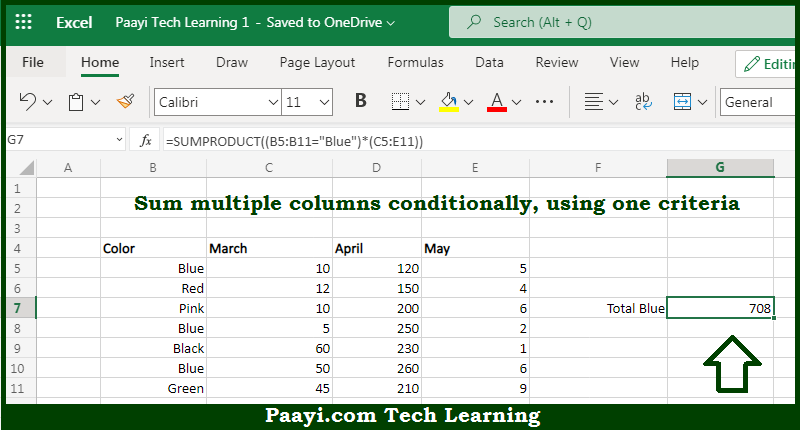# Learn How to SUM If One Criteria Applied Multiple Columns in Microsoft Excel

Written by | 0 Comments | 780 Views

In this article, you will learn how to SUM various things in Microsoft Excel using a single/combination(s) of functions. You will also know how to SUM If One Criteria Applied Multiple Columns and see the generic formula.

SUM If One Criteria Applied Multiple Columns in Microsoft Excel

The main purpose of this formula is to sum multiple columns conditionally, using one criterion. Here we will learn how to sum if one criterion is used in the multiple columns in the workbook in Microsoft Excel. That implies, with the help of a formula based on the SUMPRODUCT function you can able to sum multiple columns conditionally, using one criterion. So, with the help of this formula, you can able to sum if one criterion is used in the multiple columns in the workbook in Microsoft Excel.

General Formula to SUM If One Criteria Multiple Columns

=SUMPRODUCT((criteria_rng="red")*(sum_range))

The Explanation for the SUM If One Criteria Multiple ColumnsSo we know that with the help of the given formula above you can able to sum multiple columns conditionally, using one criterion. Here we will learn how to sum if one criterion is used in the multiple columns in the workbook in Microsoft Excel. As we know that the first expression given here in SUMPRODUCT is the criteria, checking if cells in B5:B10 contain "given value". The result is an array of TRUE FALSE values. It should be noted that the SUMPRODUCT function doesn't support wildcards. In case if you want to do a "cell contains specific text" type search, you'll need to use criteria that will return TRUE for partial matches. So, with the help of this formula, you can able to sum if one criterion is used in the multiple columns in the workbook in Microsoft Excel.